# Triangle Proportionality Theorem

Two figures are said to be similar figures if they have the same shape irrespective of their dimensions. For example, Different-sized photographs of a person, i.e. stamp size, passport size, etc., depict similar objects but are not congruent. Specific geometrical shapes or figures are always considered to be similar figures. Take an example of a circle; the shape remains the same irrespective of the change in its radius. Therefore, we can say that all the circles with different radii are similar to each other.

Similarly, all squares are always similar since the lengths of sides get altered, but the shape is always similar. In the case of similarity of triangles, the following set of conditions needs to be true for two or more triangles to be similar:

1. Corresponding angles of both the triangles are equal and
2. The corresponding sides of both the triangles are in proportion to each other.

In other words, two triangles ΔABC and ΔPQR, are similar if,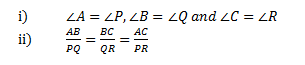Let us now discuss a fundamental theorem related to triangles known as the triangle proportionality theorem.

## Triangle Proportionality Theorem Statement

If a line is drawn parallel to any one side of a triangle so that it intersects the other two sides in two distinct points, then the other two sides of the triangle are divided in the same ratio.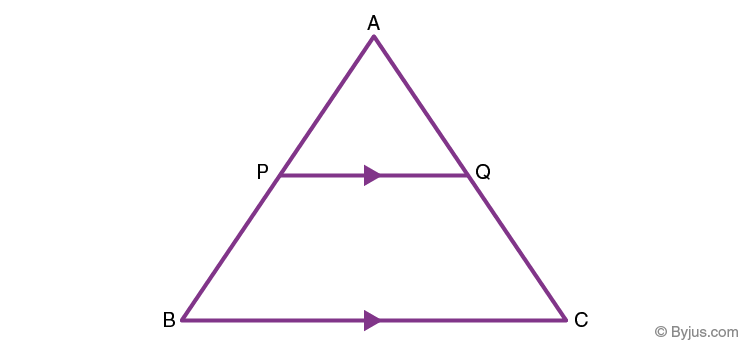Consider a triangle ΔABC as shown in the figure given above. In this triangle, if we draw a line PQ parallel to the side BC of ΔABC as shown, then according to triangle proportionality theorem, a ratio of AP to PB is equal to the ratio of AQ to QC, i.e.;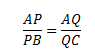Let us now try to prove this theorem.

### Proof

Given: ΔABC with line segment PQ drawn parallel to the side BC of ΔABC

Construction: Join the vertex B of ΔABC to Q and the vertex C to P to form the lines BQ and CP. Draw QN⊥ AB and PM⊥AC as shown in the given figure.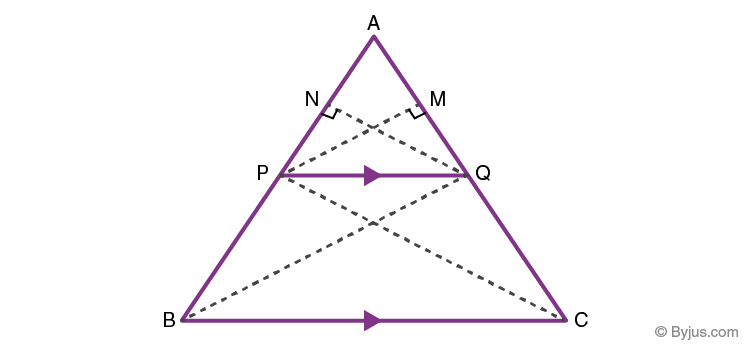Proof: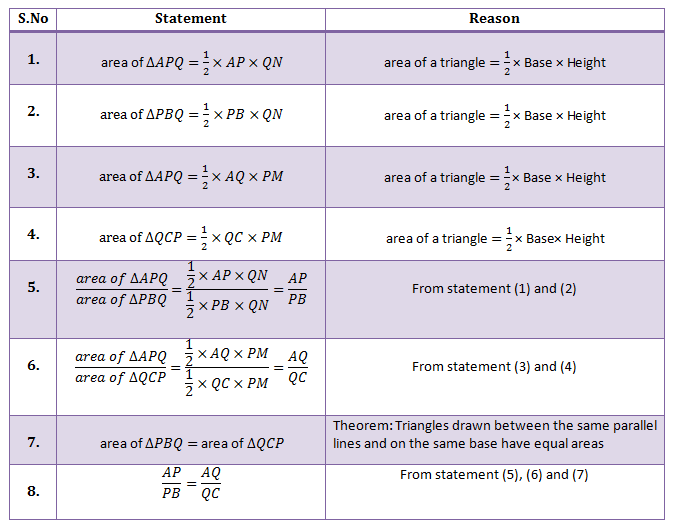Hence, the triangle proportionality theorem is proved. Also, ∆ABC and ∆APQ satisfy the required conditions for similar triangles as stated above. Therefore, it can be concluded that ∆ABC ~∆APQ.

The converse of the above theorem can also state and proved.

Let’s have a look at the example question on triangle proportionality theorem to understand how to use this theorem while solving problems on triangles.

Question:

In triangle ABC, DE || BC such that AD = 1.5 cm, DB = 3cm and AE = 1 cm. Find EC.

Solution:

Given that in triangle ABC, DE || BC.

By triangle proportionality theorem,

1.5/3 = 1/EC

EC = 3/1.5

EC = 2 cm.

## Video Lesson on BPT and Similar Triangles